# Intermediate plotting¶

### Modules - Basics¶

Last edited: December 21th 2019

## Scope of this notebook¶

This notebook can be used as reference for basic/intermediate plotting in Python using the library Matplotlib. The content will most likely be useful for the beginner and intermediate matplotlib user. We will use functions from the Python library NumPy. See this notebook for an introduction, and this module for an intermediate tutorial on NumPy. The topic Colormesh, contour, quiver, and stream plots will make use of some NumPy functions of intermediate level.

The scope of this notebook is reflected in the content list below.

## A quick review of basics¶

The code cell below summarises the plotting techniques introduced in the notebook "Basic Plotting" with comments on each line. If you want a more detailed explanation of this basic plot, see the aforementioned notebook.

In :
import matplotlib.pyplot as plt  # This command import the plotting library and aliases it as plt.
%matplotlib inline
# This is an exclusive jupyter notebook command to show the plots,
# which can be ignored if you are not working in jupyter notebook.
import numpy as np               # Import the numerical python toolkit NumPy

xValuesOfData = [-3, -2, -1, 0, 1, 2, 3]    # List of x-values
xValuesOfData = np.array(xValuesOfData)     # Convert the python list to a NumPy-array. To enable easy
# use of mathematical operations.
yValuesOfData1 = xValuesOfData**2
yValuesOfData2 = xValuesOfData**3

plt.plot(xValuesOfData, yValuesOfData1, label='$y = x^2$')
# The line above plots a 2D line (f(x) = x^2) and labels it.
# plt.plot(listOfX-valuesOfDataPoints, listOfY-valuesOfDataPoints, label=nameOfPlot)
plt.plot(xValuesOfData, yValuesOfData2, label='$y = x^3$')
plt.grid()                       # Activates grid
plt.title('A simple example')    # Sets the title of the current figure
plt.xlabel('x')                  # Set the label of the x-axis
plt.ylabel('y')                  # Sets the label of the y-axis
plt.legend()                     # Activates legend
plt.show()                       # Displays the figures (strictly not necessarry in jupyter notebook)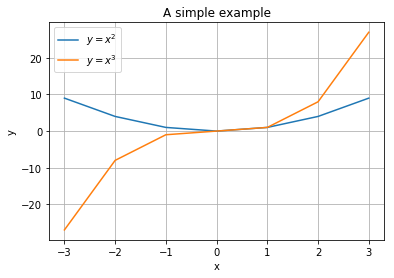## Figure formating¶

matplotlib.pyplot allows for a wide range of modification to plots and figures. In this section we will go through some of the most useful ways to help conveying your data in a desired way, and to make your plots look good.

### Style of plot¶

The default style of a plot using plt.plot is a straight line between every data point. Each plot is automatically given a unique colour, with high contrast to preceding and subsequent plots. However, you can customise the style by adding a string parameter after the data point values; plt.plot(xValues, yValues, 'style'). A specific string corresponds to a specific line/markerstyle. Below are some examples.

In :
plt.plot(xValuesOfData, yValuesOfData1, 'o')
plt.plot(xValuesOfData, yValuesOfData2, '*')
plt.plot(xValuesOfData, yValuesOfData2, ':')
plt.plot(xValuesOfData, yValuesOfData1, '--')
plt.show()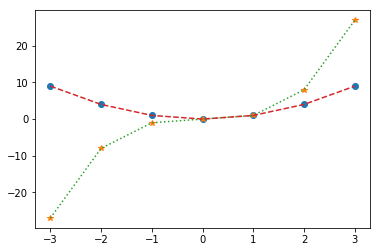Can you see which string produces which style? For a complete list of the different markers and line styles, see the documentation of matplotlib.pyplot.plot.

Colour of a plot can be selected manually by assigning the color parameter. It recognises several formats such as RGB tuples of float values (e.g. (1, 1, 1) is max red, max green and max blue, i.e. white!), hex RGB strings, or one of {'b', 'g', 'r', 'c', 'm', 'y', 'k', 'w'} (which all represents a particular color). See this link for more options.

In :
# Using a numpy function to generate an array of 100 elements uniformaly distributed from -3 to 3.
x = np.linspace(-3, 3, 100)
plt.plot(x, x**2, color=(0, 0, 0)) # RBG tuple of float values
plt.plot(x, x**3, color='#3f9faa') # Hex RBG string. #ffffff is white, #000000 is black.
plt.plot(x, x**4, color='b')       # Single character string. 'b' for blue in this case.
plt.show()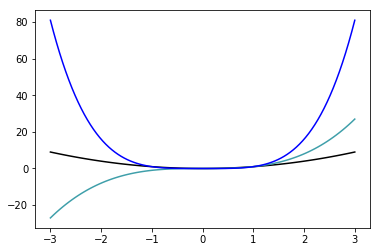Markerstyle, linestyle and colour can also be combined in one string argument. Linewidth and markersize are adjusted by assigning a float to the paramters linewidth/lw and markersize/ms, respectively.

In :
plt.plot(xValuesOfData, yValuesOfData1, 'r-*', linewidth=0.1) # Red stars with continous line in between.
plt.plot(xValuesOfData, yValuesOfData2, 'g:o', markersize=10) # Green circles with dotted line in between.
plt.show()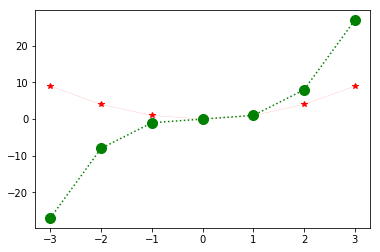### Size and resolution of figure¶

The figure size can be set by assigning a tuple of two floats to the optional parameter figsize in the function plt.figure. The two floats correspond to the width and height of the figure in inches. The resolution in terms of dots per inch (DPI), can be assigned to the optional dpi parameter. The DPI is by default 100.

In :
plt.figure(figsize=(12, 2), dpi=200) # Creates a new figure with width 12, height 2, and resolution 200 DPI.
plt.plot(x, np.sin(x))
plt.plot(x, np.cos(x + np.pi/2))
plt.show()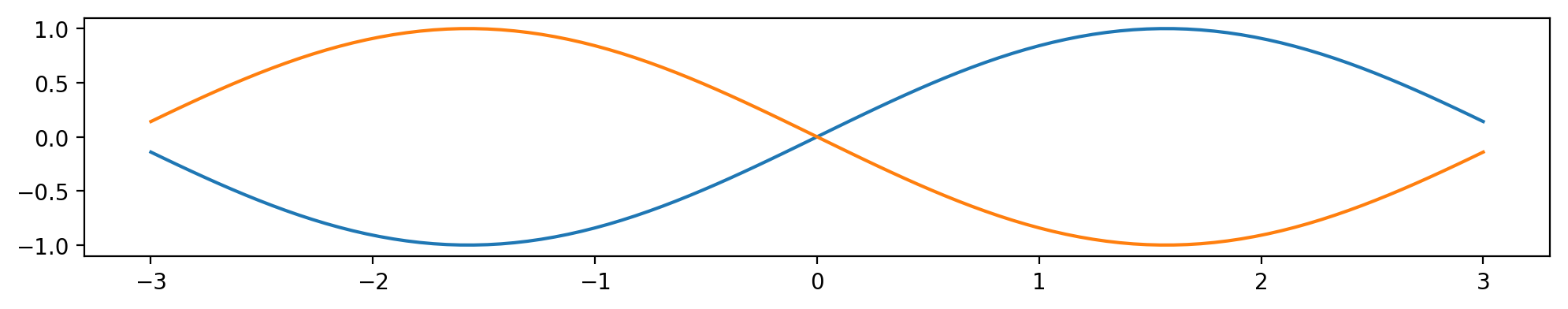### Fontsize, values and limits of axes¶

Axes limitations are set by using plt.xlimand plt.ylim.

In :
plt.figure(figsize=(12, 2), dpi=200)
plt.plot(x, np.sin(x))
plt.plot(x, np.cos(x + np.pi/2))
plt.xlim(-1, 1)       # Set the left x-axis limit to -1 and the right to 1.
plt.ylim(-0.5, 0.5)   # Set the left y-axis limit to -0.5 and the right to 0.5.
plt.show()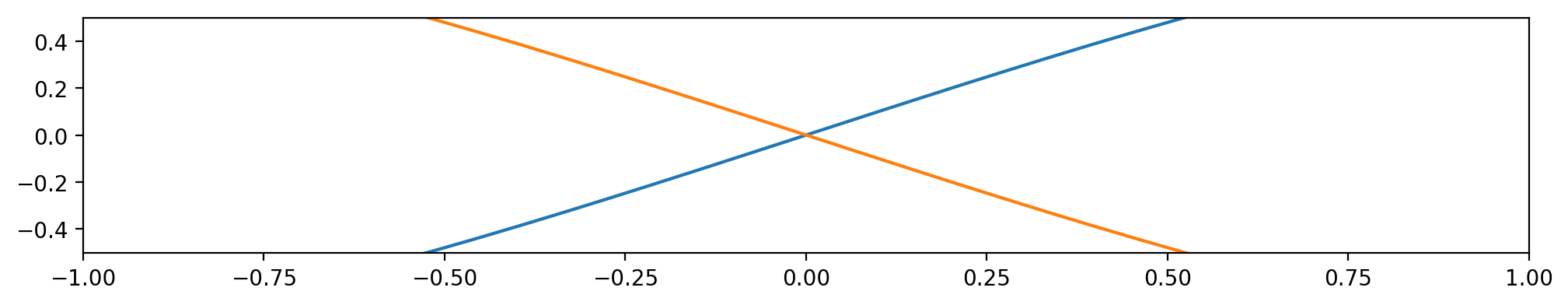Using plt.xticks and plt.yticks we can customise values on the axis. The first parameter is a list of positions at which ticks should be placed. The second parameter is optional and assigns labels to the ticks. The fontsize may be set using the optional argument fontsize. See below for an example.

In :
plt.figure(figsize=(12, 2), dpi=200)
plt.plot(x, np.sin(x))
plt.plot(x, np.cos(x))

xticks = [-np.pi/2, 0, np.pi/2]    # x-axis ticks positions
yticks = [0, 1]                    # y-axis ticks positions
xticksLabels = [r'$-\frac{\pi}{2}$', 0, r'$\frac{\pi}{2}$'] # x-axis ticks labels
yticksLabels = [0, 'Amplitude']                             # y-axis ticks positions

# Set ticks and labels
plt.xticks(xticks, xticksLabels, fontsize=20)
plt.yticks(yticks, yticksLabels)
# Activate grid, which is defined by the tick positions.
plt.grid()
plt.show()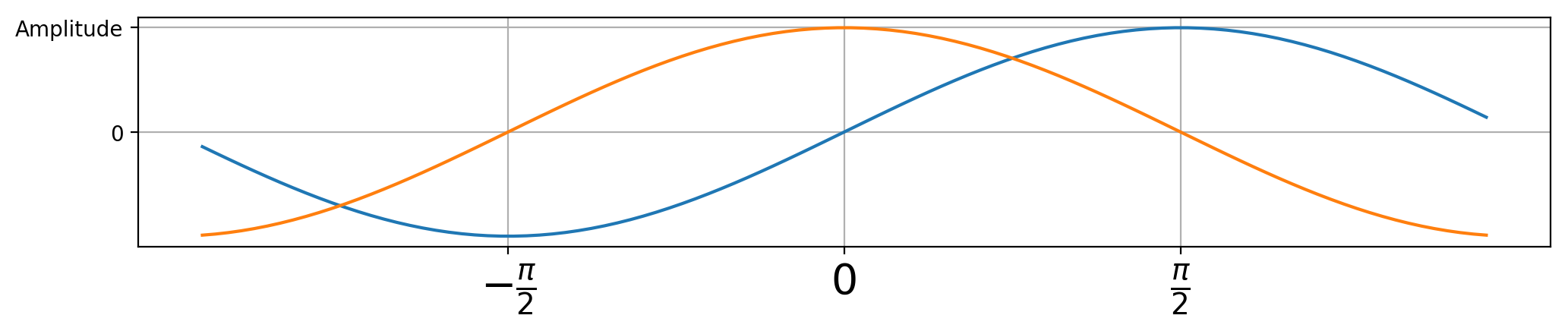### Setting common figure parameters¶

The default common figure parameters can be updated by assigning a Python dictionary to plt.rcParams.update.

In :
newParams = {'figure.figsize'  : (12, 6),  # Figure size
'figure.dpi'      : 200,      # figure resolution
'axes.titlesize'  : 20,       # fontsize of title
'axes.labelsize'  : 11,       # fontsize of axes labels
'axes.linewidth'  : 2,        # width of the figure box lines
'lines.linewidth' : 1,        # width of the plotted lines
'savefig.dpi'     : 200,      # resolution of a figured saved using plt.savefig(filename)
'ytick.labelsize' : 11,       # fontsize of tick labels on y axis
'xtick.labelsize' : 11,       # fontsize of tick labels on x axis
'legend.fontsize' : 12,       # fontsize of labels in legend
'legend.frameon'  : True,     # activate frame on lengend?
}
plt.rcParams.update(newParams) # Set new plotting parameters


For more properties to alter, see here. Updating common figure parameters is convenient to avoid writing the same code for several figures.

In :
plt.plot(x, x**3*np.sin(10*x), 'm:', label='$f(x) = x^3\sin{10x}$')
plt.plot(x, x**3, label='$f(x) = x^3$')
plt.title('An example')
plt.xlabel('$x$')
plt.ylabel('$f(x)$')
plt.legend()
plt.show()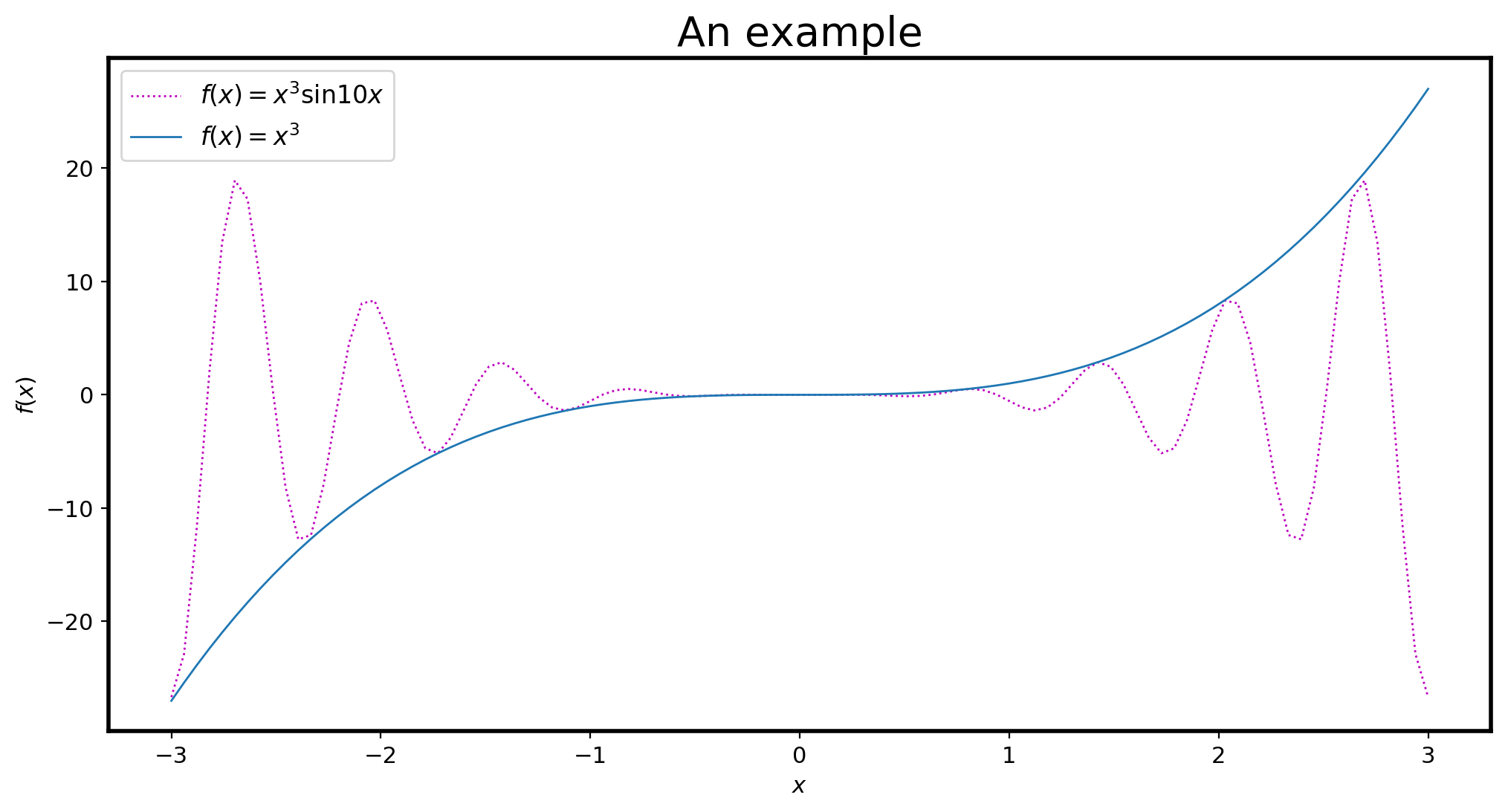### Predefined style sheets¶

Matplotlib has several predefined style sheets that changes the style and properties of the figure. They are activated by assigning a specific string to the command plt.style.use. They also provide a style sheet reference. Below are three examples.

In :
# In the code cell above, we manually updated the default parameters. Thus, we
# need to re-activate the default paramaters prior to activating a specific
# style to see how it usually appears.
plt.style.use('default')

# Activate the style sheet 'bmh'.
plt.style.use('bmh')
plt.plot(x, x**3*np.sin(10*x), ':', label='$f(x) = x^3\sin{10x}$')
plt.plot(x, x**3, label='$f(x) = x^3$')
plt.title('Style sheet "bmh"')
plt.xlabel('$x$')
plt.ylabel('$f(x)$')
plt.legend()
plt.show()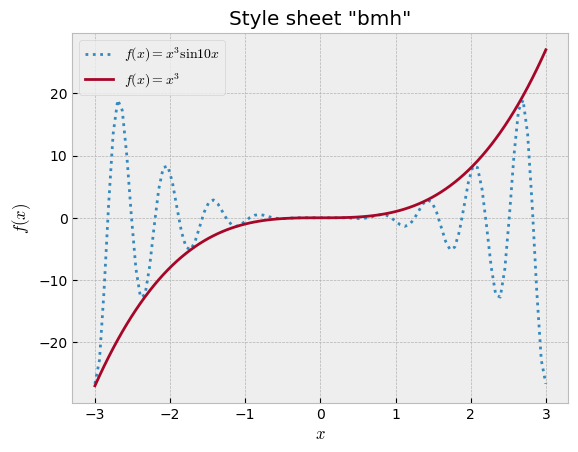In :
# Activate defualt matplotlib parameters
plt.style.use('default')
# Activate the style sheet 'dark_background'
plt.style.use('dark_background')
plt.plot(x, x**3*np.sin(10*x), ':', label='$f(x) = x^3\sin{10x}$')
plt.plot(x, x**3, label='$f(x) = x^3$')
plt.title('Style sheet "dark_background"')
plt.xlabel('$x$')
plt.ylabel('$f(x)$')
plt.legend()
plt.show()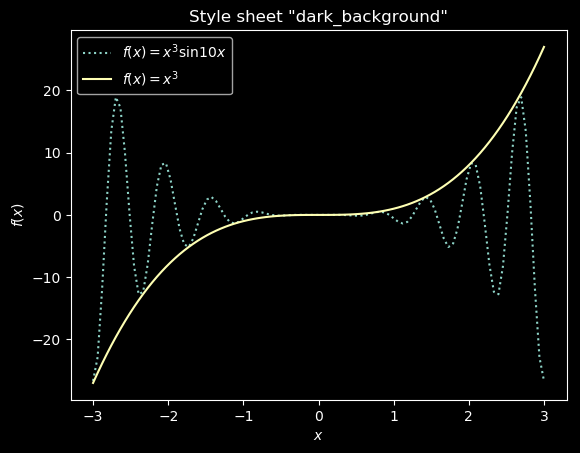In :
# Activate defualt matplotlib parameters
plt.style.use('default')
# Activate the style sheet 'classic'
plt.style.use('classic')
plt.plot(x, x**3*np.sin(10*x), ':', label='$f(x) = x^3\sin{10x}$')
plt.plot(x, x**3, label='$f(x) = x^3$')
plt.title('Style sheet "classic"')
plt.xlabel('$x$')
plt.ylabel('$f(x)$')
plt.legend()
plt.show()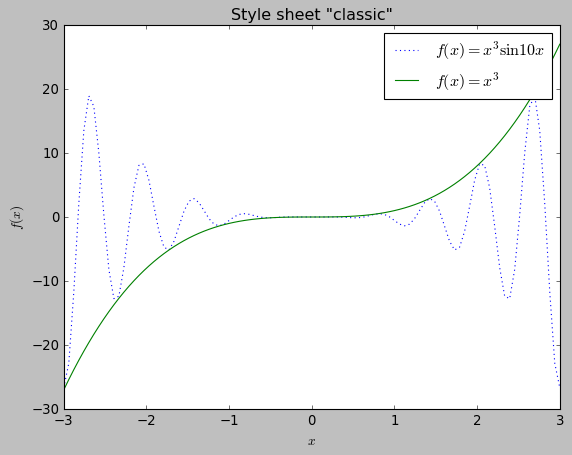## Colourmesh, contour, quiver and streamplots¶

What follows are plotting techniques particularly useful when you have a set of data points related to coordinates on a 2D grid.

### Colourmesh¶

The function plt.pcolormesh creates a rectangular grid of coloured cells. The color of each cell are specified by an input matrix $C$. Consider $$C = \begin{bmatrix} 0 & 3 & 0 \\ 1 & 2 & 1 \\ 0 & 1 & 0 \end{bmatrix}.$$

In :
# Activate defualt matplotlib parameters
plt.style.use('default')

# Create 2D-array values to be colormapped.
C = np.array([[0, 3, 0],
[1, 2, 1],
[0, 1, 0]])

plt.pcolormesh(C)
plt.colorbar()  # Adds bar to relate colour to value
plt.show()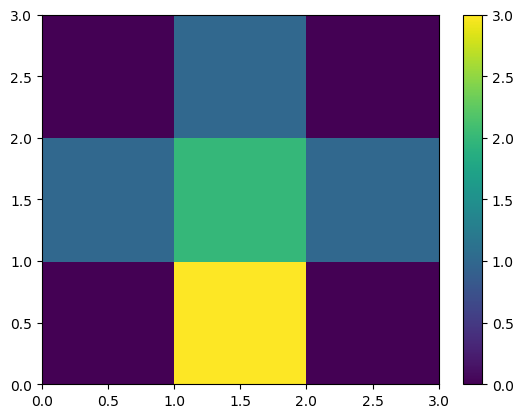Without user specification, Matplotlib defines the coordinates of the quadrilateral corners automatically. In this case they choose the "first" row of $C$, $[\text{ }0\text{ }3\text{ } 0\text{ }]$, to have the lowest $y$ values on the grid, hence the plot is flipped upside down.

Typically, $C$ is given by a function dependent on the axis values, $f = f(x, y)$. In that case we rather want to specify the range of $x$ and $y$-values, and calculate the $C$ matrix thereafter. Consider

$$f(x, y) = \sin(xy),$$

and we want to make a colour plot with the limits $x\in[0, \pi]$, $y\in[0, \pi]$.

In :
# The function f(x, y)
def f(x, y):
return np.sin(x*y)

x = np.linspace(-np.pi, np.pi, 100) # x-values
y = np.linspace(-np.pi, np.pi, 100) # y-values


However, we cannot gain the desired matrix $C$ by just sending our x and y arrays into f. This would just return a 1D array. For each element in x we need to iterate over all values in y and call f. Fortunately, NumPy has a function np.meshgrid that takes in x and y, and returns two matrices that represent this iteration.

In :
xx, yy = np.meshgrid([0, 1, 2], [0, 1, 2])
print(xx, '\n')
print(yy)

[[0 1 2]
[0 1 2]
[0 1 2]]

[[0 0 0]
[1 1 1]
[2 2 2]]


Read more about the meshgrid function in our intermediate NumPy notebook. Using this we can finally plot our colour map.

In :
xx, yy = np.meshgrid(x, y)
C2 = f(xx, yy)
plt.pcolormesh(xx, yy, C2)
plt.colorbar()
plt.title('Color plot of $\sin(xy)$')
plt.xlabel('$x$')
plt.ylabel('$y$')

Out:
Text(0, 0.5, '$y$')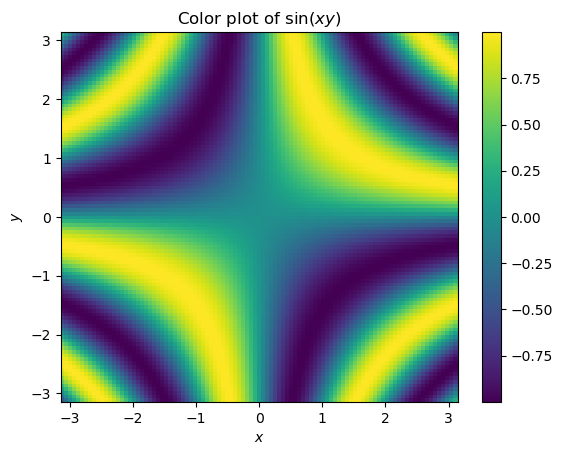If X and Y are the 2D matrices of coordinates, then the corners of cell C[i, j] and C[i, j+1] is set as in figure 1 below.Figure 1: How pcolormesh choose the corners of the color cells based on the values of the X and Y matrices.

Thus, X and Y should have one row and column more than C. If they are of equal shape, the last row and column in C will be omitted.

### Contour¶

Simply put, the function plt.contour plots contours. It draws lines where the function/matrix $f$/$C$ has the same value. plt.contour has the same parameters as for plt.pcolormesh, a 2D matrix $C$ with the values over which the lines are drawn, and optional $x$ and $y$ coordinate matrices (made using plt.meshgrid). plt.contourf draws filled contours. The optional parameter levels determine the number of contour lines drawn.

In :
# Example function g
def g(x, y):
return (x**2 + y**2)**(-1/300)

# Generate C-matrix
C3 = g(xx, yy)
# Draw contour lines
plt.contour(xx, yy, C3, 30)
plt.colorbar()
plt.show()

# Draw filled contours
plt.contourf(xx, yy, C3, 30)
plt.colorbar()
plt.show()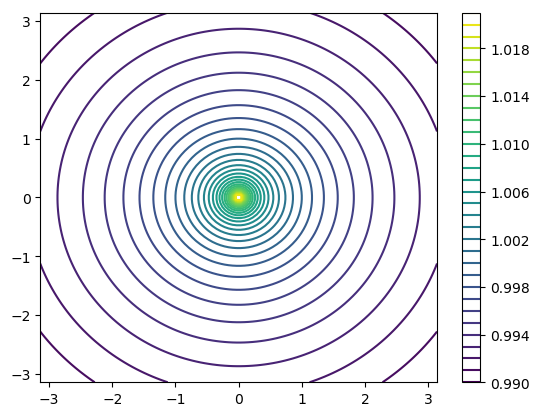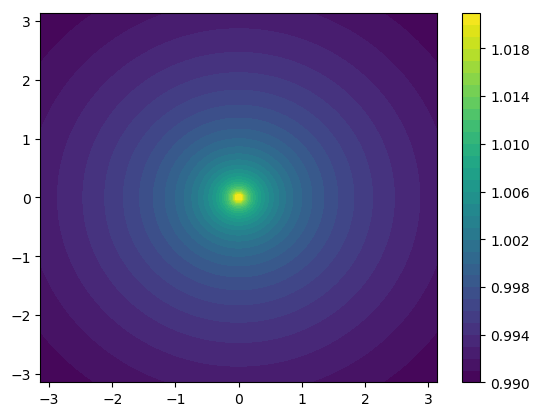### Quiver plot¶

plt.quiver is perfect if you are plotting a 2D vector field. Given the $x$ and $y$ components of the vectors it plots arrows in the corresponding direction.

In :
xComponents = np.random.rand(3, 3) # a 3x3 matrix of random number between (0, 1)
yComponents = np.random.rand(3, 3)

plt.quiver(xComponents, yComponents)
plt.show()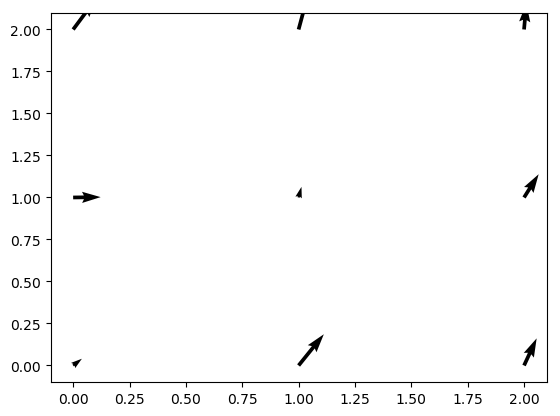The positions of the arrows can also can assigned by 2D coordinate matrices created using np.meshgrid. In addition, the arrows can be color coded using the parameter C.

In :
x2 = np.linspace(-np.pi, np.pi, 30) # x-values
y2 = np.linspace(-np.pi, np.pi, 30) # y-values
xx2, yy2 = np.meshgrid(x2, y2)      # x and y grid point coordinates

U = np.sin(xx2) # x-component of arrows
V = np.cos(yy2) # y-component of arrows
C4 = np.linalg.norm(np.array([U, V]), axis=0) # the absolute magnitude of the arrows
# Plot quivers (arrows) specified coordinates with colourcoding
plt.quiver(xx2, yy2, U, V, C4)
# Colourbar reflecting the magnitude of each colour
plt.colorbar()
plt.show()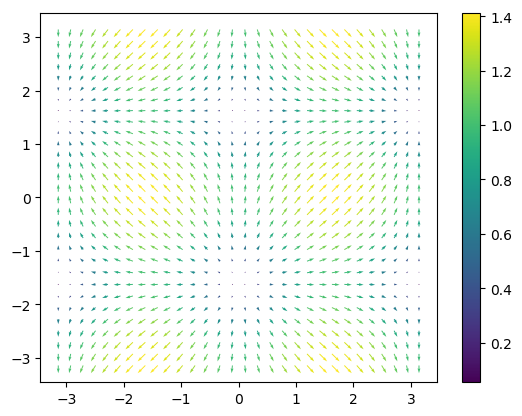### Streamplot¶

A streamplot can be used to display a 2D vector flow.

In :
xValues = np.linspace(-1, 1, 100)
yValues = np.linspace(-1, 1, 100)
xx, yy = np.meshgrid(xValues, yValues) # x and y grid point coordinates
xComponents = xx**2 + yy # Define x-components of the vectors
yComponents = xx - yy**2 # Define y-components of the vectors
plt.streamplot(xx, yy, xComponents, yComponents)
plt.show()